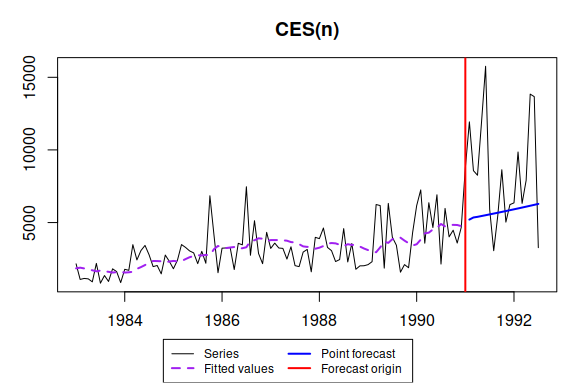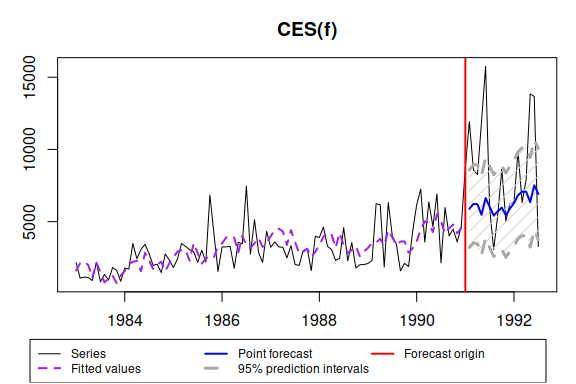# ces() - Complex Exponential Smoothing

#### 2021-09-22

This vignette covers ces() and auto.ces() functions, which are part of smooth package. In this vignette we will use data from Mcomp package, so it is advised to install it.

require(smooth)
require(Mcomp)

ces() function allows constructing Complex Exponential Smoothing either with no seasonality, or with simple / partial / full seasonality. A simple call for ces() results in estimation of non-seasonal model:

For the same series from M3 dataset ces() can be constructed using:

ces(M3$N2457$x, h=18, holdout=TRUE, silent=FALSE)
## Time elapsed: 0.04 seconds
## Model estimated: CES(n)
## a0 + ia1: 1.1085+1.0114i
## Initial values were produced using backcasting.
##
## Loss function type: likelihood; Loss function value: 840.1262
## Error standard deviation: 1419.223
## Sample size: 97
## Number of estimated parameters: 3
## Number of degrees of freedom: 94
## Information criteria:
##      AIC     AICc      BIC     BICc
## 1686.253 1686.511 1693.977 1694.567
##
## Forecast errors:
## MPE: 15.7%; sCE: 1522.5%; Asymmetry: 76.3%; MAPE: 37.3%
## MASE: 2.635; sMAE: 107.5%; sMSE: 204.4%; rMAE: 1.126; rRMSE: 1.254This output is very similar to ones printed out by es() function. The only difference is complex smoothing parameter values which are printed out instead of persistence vector in es().

If we want automatic model selection, then we use auto.ces() function:

auto.ces(M3$N2457$x, h=18, holdout=TRUE, interval="p", silent=FALSE)
## Estimating CES with seasonality: "n" "s" "f"
## The best model is with seasonality = "f"
## Time elapsed: 0.16 seconds
## Model estimated: CES(f)
## a0 + ia1: 1.1276+1.0135i
## b0 + ib1: 0.9944+1.0005i
## Initial values were produced using backcasting.
##
## Loss function type: likelihood; Loss function value: 834.4209
## Error standard deviation: 1352.622
## Sample size: 97
## Number of estimated parameters: 5
## Number of degrees of freedom: 92
## Information criteria:
##      AIC     AICc      BIC     BICc
## 1678.842 1679.501 1691.715 1693.224
##
## 95% parametric prediction interval was constructed
## 61% of values are in the prediction interval
## Forecast errors:
## MPE: 9.9%; sCE: 1235.8%; Asymmetry: 66.5%; MAPE: 33.7%
## MASE: 2.327; sMAE: 94.9%; sMSE: 167.4%; rMAE: 0.994; rRMSE: 1.135Note that prediction interval are too narrow and do not include 95% of values. This is because CES is pure additive model and it cannot take into account possible heteroscedasticity.

If for some reason we want to optimise initial values then we call:

auto.ces(M3$N2457$x, h=18, holdout=TRUE, initial="o", interval="sp")
## Time elapsed: 0.44 seconds
## Model estimated: CES(n)
## a0 + ia1: 1.0984+1.0122i
## Initial values were optimised.
##
## Loss function type: likelihood; Loss function value: 839.8342
## Error standard deviation: 1430.254
## Sample size: 97
## Number of estimated parameters: 5
## Number of degrees of freedom: 92
## Information criteria:
##      AIC     AICc      BIC     BICc
## 1689.668 1690.328 1702.542 1704.050
##
## 95% semiparametric prediction interval was constructed
## 39% of values are in the prediction interval
## Forecast errors:
## MPE: 15.2%; sCE: 1502.3%; Asymmetry: 75.7%; MAPE: 37.3%
## MASE: 2.622; sMAE: 107%; sMSE: 202.9%; rMAE: 1.12; rRMSE: 1.25

Now let’s introduce some artificial exogenous variables:

x <- cbind(rnorm(length(M3$N2457$x),50,3),rnorm(length(M3$N2457$x),100,7))

ces() allows using exogenous variables and different types of prediction interval in exactly the same manner as es():

auto.ces(M3$N2457$x, h=18, holdout=TRUE, xreg=x, xregDo="select", interval="p")
## Time elapsed: 0.51 seconds
## Model estimated: CES(f)
## a0 + ia1: 1.1276+1.0135i
## b0 + ib1: 0.9944+1.0005i
## Initial values were produced using backcasting.
##
## Loss function type: likelihood; Loss function value: 834.4209
## Error standard deviation: 1355.883
## Sample size: 97
## Number of estimated parameters: 5
## Number of degrees of freedom: 92
## Information criteria:
##      AIC     AICc      BIC     BICc
## 1678.842 1679.501 1691.715 1693.224
##
## 95% parametric prediction interval was constructed
## 61% of values are in the prediction interval
## Forecast errors:
## MPE: 9.9%; sCE: 1232.9%; Asymmetry: 66.4%; MAPE: 33.7%
## MASE: 2.325; sMAE: 94.9%; sMSE: 166.8%; rMAE: 0.993; rRMSE: 1.133